Courses

# Basic Mathematics and Measurement(Part- 1) - Physics, Solution by DC Pandey NEET Notes | EduRev

## NEET : Basic Mathematics and Measurement(Part- 1) - Physics, Solution by DC Pandey NEET Notes | EduRev

The document Basic Mathematics and Measurement(Part- 1) - Physics, Solution by DC Pandey NEET Notes | EduRev is a part of the NEET Course DC Pandey (Questions & Solutions) of Physics: NEET.
All you need of NEET at this link: NEET

Single Correct Option:
Ques 1: Percentage error in the measurement of mass and speed are 2% and 3% respectively. The error in the estimate of kinetic energy obtained by measuring mass and speed will be
(a) 12%
(b) 10%
(c) 8%
(d) 2%

Ans: 8%
Solution: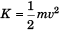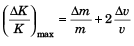∴ Maximum error = 2% + 2 (3%)
In the estimate of, kinetic energy (K) = 8%
Option (c) is correct.

Ques 2: The density of a cube is measured by measuring its mass and length of its sides. If the maximum error in the measurement of mass and length are 4% and 3% respectively, the maximum error in the measurement of density will be
(a) 7%
(b) 9%
(c) 12%
(d) 13%
Ans: 13%
Solution: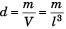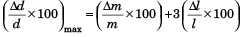= 4% + 3(3%)
= 13%
Option (d) is correct.

Ques 3: A force F is applied on a square plate of side L If the percentage error in the determination of L is 2% and that in F is 4%. What is the permissible error in pressure?
(a) 8%
(b) 6%
(c) 4%
(d) 2%
Ans: 8%
Solution: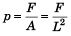∴ Permissible error in pressure (p)
= 4% + 2 (2%)
= 8%
Option (a) is correct.

Ques 4: By what percentage should the pressure of a given mass of gas be increased, so as to decrease its volume by 10% at a constant temperature?
(a) 11.1%
(b) 10.1%
(c) 9.1%
(d) 8.1%
Ans: 11.1%
Solution:  p1V1 = p2V2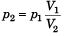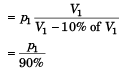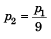∴ Percentage increase in pressure = 100/9
= 11.1
Option (a) is correct.

Ques 5: If the error in the measurement of the momentum of a particle is (+100%) Then, the error in the measurement of kinetic energy is
(a) 400%
(b) 300%
(c) 100%
(d) 200%
Ans: 200%
Solution: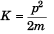∴ Error in the measurement of kinetic energy (K)
= 2 x 100%
= 200%
Option (d) is correct.

Ques 6: The number of significant figures in 3400 is
(a) 7
(b) 6
(c) 12
(d) 2
Ans: 2
Solution:
3400 = 3.400 x 103
∴ Number of significant figures = 2
Option (d) is correct.

Ques 7: The length and breadth of a metal sheet are 3.124 m and 3.002 m respectively. The area of this sheet upto correct significant figure is
(a) 9.378 m2
(b) 9.37 m2
(c) 9.378248 m2
(d) 9.3782 m2
Ans: 9.378 m2
Solution:
A = 3.124 m x 3.002 m
= 9.378/248 m2
= 9.378 m2
Option (a) is correct.

Ques 8: Let g be the acceleration due to gravity at earth’s surface and K the rotational kinetic energy of the earth. Suppose the earth’s radius decreases by 2%. Keeping all other quantities constant, then
(a) g increases by 2% and K increases by 2%
(b) g increases by 4% and K increases by 4%
(c) g decreases by 4% and K decreases by 2%
(d) g decreases by 2% and K decreases by 4%
Ans: g increases by 4% and K increases by 4%
Solution: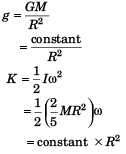∴ Decrease in R (radius) by 2% world increase g by 4% and decrease K (rotational kinetic energy) by 4%.
Option (b) is correct.

Ques 9: The heat generated in a circuit is dependent upon the resistance, current and time for which the current is flown. If the error in measuring the above are 1%, 2% and 1% respectively. The maximum error in measuring the heat is
(a) 8%
(b) 6%
(c) 18%
(d) 12%
Ans: 6%
Solution:
Heat (H) = I2 Rt
∴ Maximum error in measuring heat (H)
=2 (2%) + 1% + 1%
= 6%
Option (b) is correct.

Ques 10: The length, breadth and thickness of a block are given by / = 12 cm, b = 6 cm and t =2.45 cm. The volume of the block according to the idea of significant figures should be
(a) 1 x 102 cm3
(b) 2 x 102 cm3
(c) 1.763 x 102 cm3
(d) None of these

Ans: 2 x 102 cm3
Solution:
V = lbt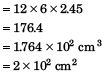Option (b) is correct.

Ques 11: If separation between screen and point source is increased by 2%. What would be the effect on the intensity?
(a) increases by 4%
(b) increases by 2%
(c) decreases by 2%
(d) decreases by 4%
Ans: decreases by 4%
Solution: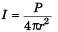i.e., Ir2 = constant
i.e., if 'r' is increased by 2%,
the intensity will decrease by 4%.
Option (d) is correct.

Ques 12: The significant figures in the number 6.0023 are
(a) 2
(b) 5
(c) 4
(d) 1
Ans: 5
Solution:
Option (b) is correct.

Ques 13: If error in measurement of radius of a sphere is 1%, what will be the error in measurement of volume?
(a) 1%
(b) 1/3 %
(c) 3%
(d) 10%
Ans: 3%
Solution: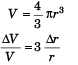= 3(1%)
= 3%
Option (c) is correct.

Ques 14: The volume of a cube in m3 is equal to the surface area of the cube in m2. The volume of the cube is
(a) 64 m3
(b) 216 m3
(c) 512 m3
(d) 196 m3
Ans: 216 m3
Solution:
a3 = 6a2 (given)
∴ a = 6
⇒ V = 63 = 216 m3
Option (b) is correct.

Ques 15: The length of a simple pendulum is about 100 cm known to have an accuracy of 1 mm. Its period of oscillation is 2 s determined by measuring the time for 100 oscillations using a clock of 0.1 s resolution. What is the accuracy in the determined value of g?
(a) 0.2%
(b) 0.5%
(c) 0.1%
(d) 2%
Ans: 0.2%
Solution: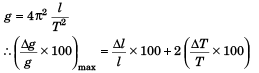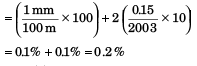Option (a) is correct.

Ques 16: Charge on the capacitor is given by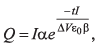where α and β are constants, t = time, I = current, ΔV = potential difference. Then dimensions of β/α is same as the dimensions of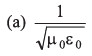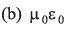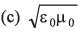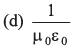Ans:Solution: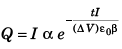(given)
We know that
Q = It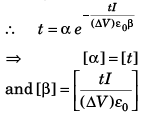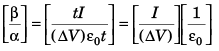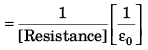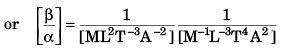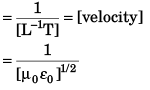Option (a) is correct.

Ques 17: A physical quantity A is dependent on other four physical quantities p, q, r and s as given by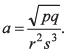The percentage error of measurement in p , q , r and s are 1%, 3%, 0.5% and 0.33% respectively, then the maximum percentage error in A is
(a) 2%
(b) 0%
(c) 4%
(d) 3%

Ans: 4%
Solution: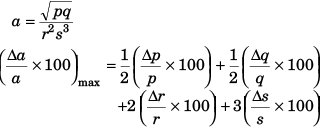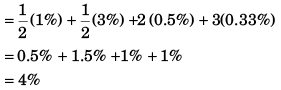Option (c) is correct.

Ques 18: The distance moved by the screw of a screw gauge is 2 mm in four rotations and there are 50 divisions on its cap. When nothing is put between its jaws, 30th division of circular scale coincides with reference line, with zero of circular scale lying above the reference line. When a plate is placed between the jaws, main scale reads 2 divisions and circular scale reads 20 divisions. Thickness of plate is
(a) 0.9 mm
(b) 1.2 mm
(c) 1.4 mm
(d) 1.5 mm
Ans: 1.5 mm
Solution: Least count of main scale
= 2mm/4 = 0.5 mm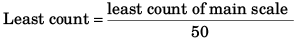= 0.1 mm Zero error = - 30 x 0.01 mm = -0.3 mm
(–ive sign, zero of circular scale is lying above observed reading of plate thick)
= 2 MSR + 20 CSR
= (2 × 0.5 mm) + (20 × 0.01 mm) = 1 mm + 0.2 mm = 1.2 mm.
= observed reading - zero error = 1.2 mm + 0.3 mm = 1.5 mm
Option (d) is correct.

More than One Correct Options:

Ques 1: Given,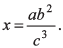If the percentage errors in a, b and c are + 1%, ± 4% and + 2% respectively.
(a) The percentage error in x can be +13%
(b) The percentage error in x can be +7%
(c) The percentage error in x can be +18%
(d) The percentage error in x can be +19%
Ans: (a, b)
Solution:
Maximum percentage error in x:
= 1 (% error in a) + 2 (% error in b) +3 (% error in c)
= 15%

Assertion and Reason:
Directions: Choose the correct option.
(a) If both Assertion and Reason are true and the Reason is correct explanation of the Assertion.
(b) If both Assertion and Reason are true but Reason is not the correct explanation of Assertion.
(c) If Assertion is true, but the Reason is false.
(d) If Assertion is false but the Reason is true.
Assertion: A screw gauge having a smaller value of pitch has greater accuracy.
Reason: The least count of screw gauge is directly proportional to the number of divisions on circular scale.

Ans: If Assertion is true, but the Reason is false.
Solution:
Least count of  screw gauge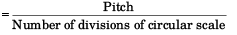Less the value of pitch, less will be least count of screw gauge leading to len uncertainty that is more accuracy in the measurement.
Thus, assertion is true.
From the above relation we conclude that least count of  screw gauge is inversely proportional to the number of divisions of circular scale.
Thus reason is false.
Option (c) is correct.

Match the Columns
Ques 1: Match the following two columns.

 Column-I Column-II (a) GMeMs (p) [M2L2T-3]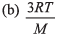(q ) [ML3T-2]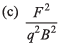(r) [L2T-2]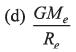(s) None

Ans: (a) → q (b) → r (c) → r (d) → r
Solution: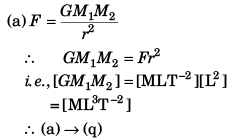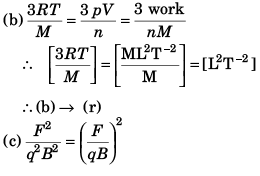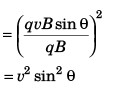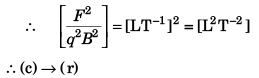(d)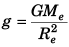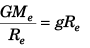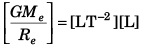= [L2 T–2]
∴ (d) → (r)

Offer running on EduRev: Apply code STAYHOME200 to get INR 200 off on our premium plan EduRev Infinity!

210 docs

,

,

,

,

,

,

,

,

,

,

,

,

,

,

,

,

,

,

,

,

,

,

,

,

;